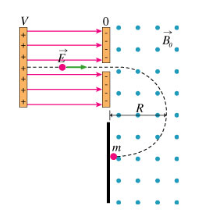# Problem: J. J. Thomson is best known for his discoveries about the nature of cathode rays. Another important contribution of his was the invention, together with one of his students, of the mass spectrometer. The ratio of mass m to (positive) charge q of an ion may be accurately determined in a mass spectrometer. In essence, the spectrometer consists of two regions: one that accelerates the ion through a potential difference V and a second that measures its radius of curvature in a perpendicular magnetic field. (Figure 1)The ion begins at potential V and is accelerated toward zero potential. When the particle exits the region with the electric field it will have obtained a speed u.Part AWith what speed u does the ion exit the acceleration region?Find the speed in terms of m, q, V, and any constants.Part BAfter being accelerated, the particle enters a uniform magnetic field of strength B0 and travels in a circle of radius R (determined by observing where it hits on a screen-as shown in the figure). The results of this experiment allow one to find m/q in terms of the experimentally measured quantities such as the particle radius, the magnetic field, and the applied voltage.What is m/q?Express m/q in terms of B0, V, R, and any necessary constants.

###### FREE Expert Solution

(a)

Electric potential energy:

$\overline{){\mathbf{U}}{\mathbf{=}}{\mathbf{q}}{\mathbf{V}}}$

Kinetic energy:

$\overline{){\mathbf{K}}{\mathbf{E}}{\mathbf{=}}\frac{\mathbf{1}}{\mathbf{2}}{\mathbf{m}}{{\mathbf{v}}}^{{\mathbf{2}}}}$

The electron acquires kinetic energy equal to the potential energy.

84% (450 ratings)###### Problem Details

J. J. Thomson is best known for his discoveries about the nature of cathode rays. Another important contribution of his was the invention, together with one of his students, of the mass spectrometer. The ratio of mass m to (positive) charge q of an ion may be accurately determined in a mass spectrometer. In essence, the spectrometer consists of two regions: one that accelerates the ion through a potential difference V and a second that measures its radius of curvature in a perpendicular magnetic field. (Figure 1)

The ion begins at potential V and is accelerated toward zero potential. When the particle exits the region with the electric field it will have obtained a speed u.

Part A

With what speed u does the ion exit the acceleration region?

Find the speed in terms of m, q, V, and any constants.

Part B

After being accelerated, the particle enters a uniform magnetic field of strength B0 and travels in a circle of radius R (determined by observing where it hits on a screen-as shown in the figure). The results of this experiment allow one to find m/q in terms of the experimentally measured quantities such as the particle radius, the magnetic field, and the applied voltage.
What is m/q?

Express m/q in terms of B0, V, R, and any necessary constants.